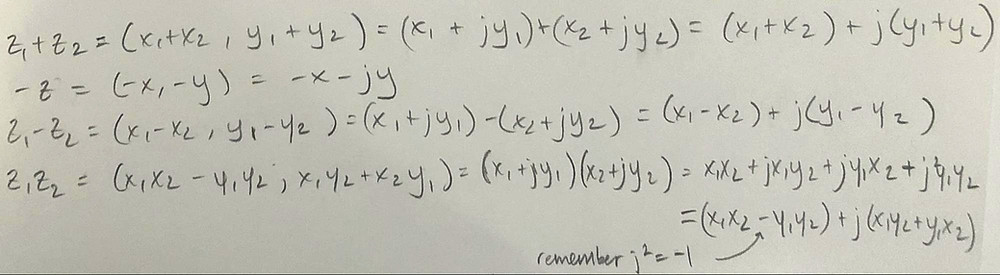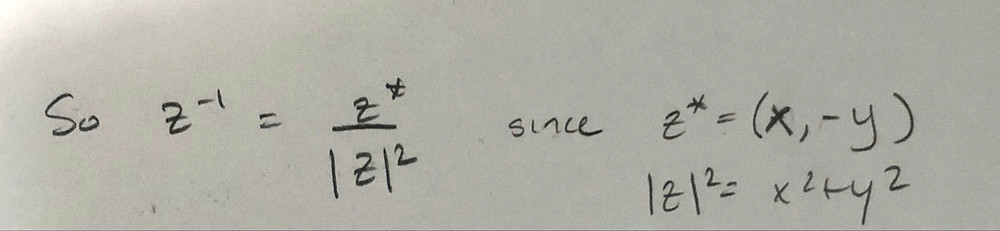# Imaginary Numbers and Euler’s Formulas Review

Updated: Jan 10, 2020

A lot of people seem to freak out when they see an i in math or j in electrical engineering. So hopefully this will help.

The first thing we want to go over is what i and j even are.The other aspect of imaginary numbers that will help us understand how they are used in electrical engineering (and systems/signaling in general) is that they can be graphed on a complex plane.NOTE: You may have also noticed that when we were graphing z = (x,y) on the complex plane we formed a right triangle. So:Also:Looking at imaginary numbers like this, we can also see how they can be added, subtracted, and multiplied like any other numbers.There are also a couple of new operations:You may have noticed that I did not include division, and multiplication is a little difficult as well. For this, we will use Euler’s formulas.The four underlined formulas are the common forms of Euler’s formulaMultiplying and dividing exponentials is a lot easier than multiplying and dividing complex numbersIf we were asked to change that back into a complex number, then:These are a few more useful forms of euler’s formulas:These techniques are most useful in electrical engineering when dealing with AC circuits, or in other words, circuits with sinusoidal inputs## Shayan Raofi

January 10, 2020

View Profile

#### Get in Touch

Thanks! We'll reach out shortly.